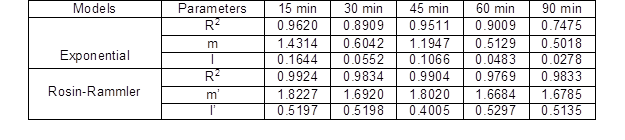Duan, W., Liu, Z., Liu, P., and Hui, L. (2018). "Estimation of acid-hydrolyzed cellulose fiber size distribution with exponential and Rosin-Rammler (R-R) laws," BioRes. 13(4), 7792-7804.

#### Abstract

A new method was developed to characterize the cellulose fiber (hardwood dissolving pulp) size distribution after 50 wt% sulfuric acid hydrolysis at different hydrolysis temperatures with exponential and Rosin-Rammler (R-R) laws. The results show that the two above laws can be effective to explain the cellulose hydrolysis process. The exponential and the R-R laws were found to be well fitted to the sulfuric acid hydrolyzed cellulose fiber length distribution. The cumulative number distribution from Fiber Tester was shown to fit the exponential distribution well, while the cumulative mass distribution obtained from the acid hydrolysis was found to be suitable to the R-R law via an approximation process. The results from SEM analyses also supported the above conclusions. This approach can be used to characterize the cellulosic fiber properties before a further process of biorefinery, nanocellulose preparation, or other application of lignocellulosic fibers.

Estimation of Acid-Hydrolyzed Cellulose Fiber Size Distribution with Exponential and Rosin-Rammler (R-R) Laws

Weijiang Duan,a Zhong Liu,a,* Pengtao Liu,a and Lanfeng Hui a,b

A new method was developed to characterize the cellulose fiber (hardwood dissolving pulp) size distribution after 50 wt% sulfuric acid hydrolysis at different hydrolysis temperatures with exponential and Rosin-Rammler (R-R) laws. The results show that the two above laws can be effective to explain the cellulose hydrolysis process. The exponential and the R-R laws were found to be well fitted to the sulfuric acid hydrolyzed cellulose fiber length distribution. The cumulative number distribution from Fiber Tester was shown to fit the exponential distribution well, while the cumulative mass distribution obtained from the acid hydrolysis was found to be suitable to the R-R law via an approximation process. The results from SEM analyses also supported the above conclusions. This approach can be used to characterize the cellulosic fiber properties before a further process of biorefinery, nanocellulose preparation, or other application of lignocellulosic fibers.

Keywords: Fiber length distribution; Sulfuric acid hydrolysis; Exponential law; Rosin-Rammler law

Contact information: a: Tianjin Key Laboratory of Pulp and Paper, Tianjin University of Science and Technology, Tianjin, 300457, China; b: State Key Laboratory of Pulp and Paper Engineering, South China University of Technology, Guangzhou, 510641, China; *Corresponding author: mglz@tust.edu.cn

INTRODUCTION

Cellulose, the most abundant bioresource in nature, is the most important source for the preparation of related down-stream products, such as glucose (Sakdaronnarong et al. 2018), disaccharides (Kwan et al. 2018), oligosaccharides (Wang et al. 2017), microcrystalline cellulose (MCC) (Wu et al. 2017), and cellulose nano-crystals (CNC) (Yin et al. 2017) etc. It is well known that most of the products mentioned above are produced via an acid hydrolysis process. Sulfuric acid is one type of high-efficiency, low-cost solvent and catalyst that has been commonly used to hydrolyze cellulose fibers to obtain the desired products. For example, Gårdebjer et al. (2015) obtained CNC colloid suspensions via a 64 wt% sulfuric acid hydrolysis. Dussán et al. (2014) subjected sugarcane bagasse cellulose to 2 wt% sulfuric acid hydrolysis to produce glucose or disaccharide solutions. Thus, how to characterize or evaluate the properties of bioresources, such as fiber length distribution following sulfuric acid hydrolysis, is a relevant topic for study.

Many reports have been published on obtaining cellulose fiber length or studying fiber length distribution with various methods. For example, Hertel and Zervigon (1936) first proposed a theory that used an indirect fibrograph method to determine cotton fiber length from a non-mathematical point of view. Zeidman et al. (1991) investigated cotton fibers and employed the short fiber content as a parameter to describe the range and shape of cotton fiber length distribution. Tasman (1972) reported that the fiber length distribution of white birch was approximately in line with Gauss’s law. Yan (1975) and Kropholler and Sampson (2001) found that the length distribution of papermaking cellulose fibers followed a log-normal distribution. Krifa (2008) found that cotton fiber length distributions conformed to a mixture of two Weibull probability density functions. Kuang et al. (2015) also reported that the multimodal mixed Weibull distribution was well-fitted to the yarn fiber length density function when used to predict fiber unevenness. It has been reported that size distribution data are most commonly presented as log-probability plots of diameter versus the cumulative volume percent oversize, which give straight line plots if the distribution is log-normal (Smith and Jordan 1964).

In addition, it has been evidenced from the study of Morris and Warburton (1984) that the curve fitting of size and size distributions of solid particles (0.01 to 100 μm diameter), such as three-walled w/o/w microcapsules, can be used to model size-frequency plots with distribution equations, for example, exponential and Rosin-Rammler laws. However, it has not been reported to estimate the cellulose fiber length distribution after sulfuric acid hydrolysis with the two aforementioned log-normal equations.

In this study, a new method to estimate the cellulose fiber length distribution following 50 wt% sulfuric acid hydrolysis with exponential and R-R laws was proposed. Two issues in this study should be addressed beforehand: 1) why a 50 wt% concentration of sulfuric acid in this research was selected; and 2) why exponential and R-R laws were applied in the paper to estimate the cellulose fiber length distribution.

As for the first issue, the selection of 50 wt% sulfuric acid concentration was based on previous research. Barbash et al. (2016) found that when the acid concentration was higher than 50 wt% in the hydrolysis process, the sulfuric acid destroys both the amorphous and crystalline regions of cellulose, which would be hydrolyzed into glucose absolutely. In contrast, the sulfuric acid molecules in a weak sulfuric acid concentration (less than 50 wt%) could not penetrate into the inner core of cellulose fibers, and then insufficient hydrolysis could lead to a heterogeneous cellulose fiber suspension that would negatively impact the experimental results (Chang et al. 2010). Based on the discussion above, the selected sulfuric acid concentration (50 wt%) was deemed a reasonable choice for this study.

As for the second issue, in general, two methods were mainly used to characterize the rock fragmentation: statistical and physical (Elek and Jaramaz 2005) methods. In statistics, the rock fragmentation model by Turcotte (1986) was based on an exponential relationship between the particle size and the cumulative number of rocks. Furthermore, rock fragmentation not only involves a statistical problem, but also a physical process. The 2-parameter Weibull distribution, which is also called the Rosin-Rammler (R-R) distribution, is usually utilized to represent the rock particle size distributions generated by grinding, milling, and crushing operations (Rosin and Rammler 1933). In addition, the R-R law was used to describe the particle size distribution of incineration bottom ash (Farizhandi et al. 2016). The R-R equation has been used to describe the particle size distribution of powders. Thus, the particle size was substituted with fiber length in this study. The cumulative exponential distribution, as well as the cumulative R-R distribution, were applied to predict the cellulose fiber number. This study is to put forward a new method to estimate the cellulose fiber length distribution following 50 wt% sulfuric acid hydrolysis with exponential and R-R laws.

EXPERIMENTAL

Materials

Hardwood dissolving pulp, obtained from Shandong Sun Paper Industry Joint Stock Co., Ltd. (Shandong, China), was used as the raw material. This pulp has a moisture content of 5%, α-cellulose content of over 95%, and the average degree of polymerization (DP) of about 700. The pulp fibers were subjected to grinding process and followed by passing through 5,000 mesh screen. Other chemical reagents, such as sulfuric acid (98 wt%, AR-grade) and sodium bicarbonate (AR-grade) were purchased from Sigma-Aldrich (Shanghai, China) and used without further purification.

Methods

Acid hydrolysis

Sulfuric acid (98 wt%) was first carefully diluted to 50 wt% with deionized water. Dissolving-grade pulps fibers (1 g, air-dried) were then placed into a beaker, followed by the addition of the as-prepared 50 wt% H2SO4 under continuous magnetic stirring to disperse the cellulose fibers well. The acid:cellulose ratio was 50:1 (g/mL) according to the previous report (Fortunati et al. 2013). The reaction systems were then subjected to a thermostatic control unit at 50 °C, 60 °C, and 70 °C, individually, and the hydrolysis process duration sets tested individually were 15 min, 30 min, 45 min, 60 min, and 90 min. At the end of the reaction, the resulting suspensions were diluted with 10 times cold deionized water to terminate the reaction. Then, the obtained suspension was centrifuged and neutralized with a NaHCO3 solution for further analysis.

Fiber length measurements

Subsequently, the cellulose fiber length of the control and treated samples were measured using a L&W Fibers Tester (ABB Lorentzen & Wettre, Kista, Sweden) based on image analysis. All of the tests were conducted three times for each sample, and the average of all three was reported.

Scanning electron microscope (SEM) analyses of cellulose fiber

Cellulose fiber morphology derived from different sulfuric acid hydrolysis was investigated by SEM micrographs recorded with a JEOL JSM 6390 LA scanning electron microscope (JEOL JSM 6390, Tokyo, Japan).

Exponential distribution

The exponential distributions of the control and treated samples were obtained via the exponential law, as shown in Eq. 1,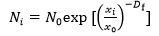(1)

where Ni is the number of fragmented elements of length xi at the ith iteration step, N0 is the number of initiators of length x0 at = 0, and Df is a free parameter.

The cumulative form of the exponential law can be used for fitting the data of the cumulative length distribution, as shown in Eq. 2: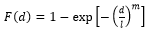(2)

Finally, the linear exponential function can be obtained by a mathematical transformation of Eq. 2, as shown in Eq. 3: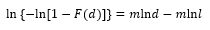(3)

Rosin-Rammler distribution

The empirical R-R distribution function is given below, as shown in Eq. 4,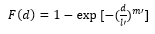(4)

where F(d) denotes the cumulative mass distribution, describing the fraction of fibers shorter than d, while d represents the fiber length (mm), and m’ is the spread of fiber length, a dimensionless parameter.

The scale parameter l’ is the fiber length. Shape parameter m’ indicates the breadth of the fiber length distribution. These two parameters can be determined by plotting the cumulative form of ln-ln coordinates by taking the natural logarithm of Eq. 1 twice through linear regression to obtain Eq. 5: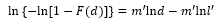(5)

The cumulative mass distribution in the R-R equation could not be replaced by cumulative number distribution (Azzouz et al. 2008). Considering that mass distribution cannot be obtained through optical measurement, approximate processing was necessary (Ring and Bacon 1997). The total weight (w) of one sample with a uniform length could be expressed through the product of number, length, and coarseness (coarseness from the same fiber resource could be taken as a constant, coarseness Q is the mass of the fiber per unit length, in g/m). After the approximate treatment, the corresponding distribution was transformed into the mass-weighted distribution. The fiber mass of each length grade is described by Eq. 6,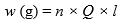(6)

where w is the total weight of one sample with a uniform length (g), n is number of sample fibers, Q is fiber coarseness (g/m), and l is the uniform fiber length (m). Thus, the cumulative mass distribution data for the R-R fitting can be obtained.

RESULTS AND DISCUSSION

TEM Analyses of Cellulose Fibers from Sulfuric Acid Hydrolysis

TEM analyses were conducted to investigate the morphology of cellulose fibers derived from the control sample and sulfuric acid hydrolysis samples, as shown in Fig. 1. Fig. 1(a) exhibits the cellulose fiber shape without sulfuric acid hydrolysis; it was shown that cellulose fiber possessed intact structure with fiber length of ca. 2 μm and width of ca. 0.1 to 0.2 μm. The fiber structure changed a little when sulfuric acid hydrolysis was applied at 50 °C for 15 min; however, the length of fiber decreased from ca. 2 μm to 1 μm, as shown in Fig. 1(b), and the width decreased to ca. 0.1 μm. This indicated that sulfuric acid hydrolysis at low temperature and short hydrolysis duration has more effects on the length of cellulose fiber. It was noted that the fiber structure was destroyed by acid hydrolysis when the acid hydrolysis duration was extended to 45 min, as shown in Fig. 1(c). The surface structure of cellulose fiber was etched and hydrolyzed by sulfuric acid when the temperature increased from 50 °C to 60 °C for 15 min hydrolysis duration, as shown in Fig. 1(d), in which most of cellulose fibers had a length of less 0.5 μm, yet the width changed little compared that of Fig. (c). This demonstrated that hydrolysis temperature has a stronger effect than hydrolysis duration. Fig. 1(e) and (f) are both from acid hydrolysis at 70 °C, and for 15 min and 60 min separately. It was shown that the fiber morphology changed a lot once the temperature increased to 70 °C, and that most of the fiber length decreased to less 0.5 μm, while the width may decrease to less 0.1 μm. The hydrogen bonds between cellulose fibers were hydrolyzed, which exposed more free hydroxyl groups on the surface of fibers. It was also shown that some fiber debris were aggregated into chunks due to the small size by hydrogen bonding, as shown in Fig. 1(f), which reduced the length-width ratio of cellulose fibers.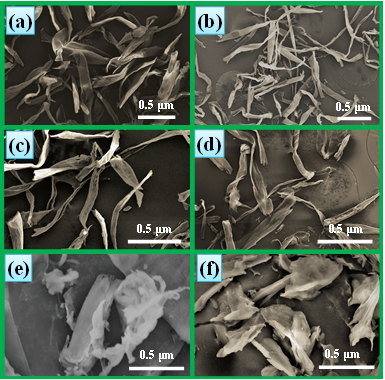Fig. 1. TEM images of cellulose fibers derived from (a) control sample and sulfuric acid hydrolysis samples ((b) at 50 °C for 15 min; (c) at 50 °C for 45 min; (d) at 60 °C for 15 min; (e) at 70 °C for 15 min; (f) 70 °C for 60 min)

Number-weighted Cellulose Fiber Length Distribution

Figure 1 shows the number-weighted length distribution of cellulose fibers. As shown in Fig. 1(a), the untreated cellulose fibers had a normal distribution that showed the minimum length of the control sample was approximately 0.25 μm, while the maximum length was 2.0 μm. The median length was approximately 0.8 μm.

However, the number-weighted length distributions were not normal curves after the cellulose fibers were subjected to sulfuric acid hydrolysis, as shown in Figs. 1(b), (c), and (d). The trend curves of length distribution became lower and wider and shifted to the left compared to Fig. 1(a). From Fig. 1 it was concluded that: 1) all of the lengths of these treated fibers were less than 1 μm when the hydrolysis duration was 15 min; 2) the length of the treated fibers became increasingly shorter as the hydrolysis duration increased; 3) the treated fibers had a narrower length distribution compared with that of the control; and 4) the length of the treated fibers was increasingly shorter as the hydrolysis temperature increased, and almost 95% of the treated fibers had a length of approximately 0.25 μm when the hydrolysis temperature was 70 °C and the duration was 90 min, as shown in Fig. 1(d).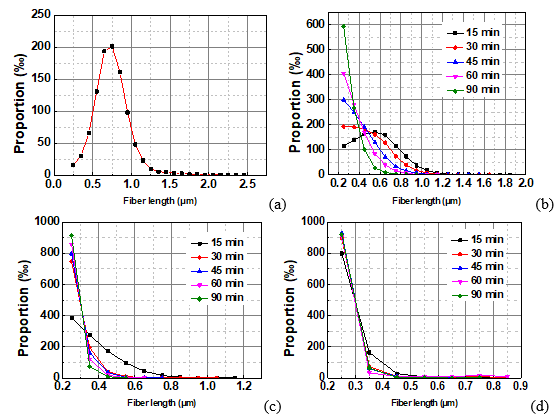Fig. 1. Number-weighted length distribution of (a) untreated cellulose fibers and treated cellulose fibers with sulfuric acid hydrolysis at (b) 50 °C, (c) 60 °C, and (d) 70 °C

Figure 2 shows the exponential fitting to the number-weighted length distribution of cellulose fibers, according to Eqs. 1, 2, and 3. The data shown in Fig. 2 have been listed in Tables S1, S2, S3, and S4 in the Supporting Information (see Appendix). Figure 2(a) shows the exponential fitting curve to number-weighted length distribution of untreated cellulose fibers. It shows a cellulose length of 1 μm (ln(x) = 0) as the break point dividing the two straight lines. It was demonstrated that both the longer part (≥ 1 mm) and the shorter part (≤ 1 mm) of the untreated cellulose fibers fit the exponential distribution well, which corresponded with the results seen in Fig. 1(a) and Table S1. Thus, the fiber length of untreated cellulose obeyed the exponential distribution and it was estimated via an exponential curve.

Figure 2(b) shows the exponential fitting to number-weighted length distribution of treated cellulose fibers with sulfuric acid hydrolysis at 50 °C. Most of the length of the treated cellulose fibers was less than 1 μm, as shown in Fig. 1(b). It can be noted in Fig. 2(b) that the number-weighted length distribution (experimental data) was well fitted to the theoretical lines decided by exponential law, which indicated that it was reasonable to estimate the cellulose fiber length distribution with an exponential law. As shown in Table S2 and Fig. 2(b), the values of R2decreased from 0.9999 to 0.9880 as the acid hydrolysis duration increased from 15 min to 90 min, which indicated that as the acid hydrolysis reaction progressed, the degree of suitability between exponential law and the fiber length distribution was reduced, which was ascribed to the reduction of cellulose fiber length as the reaction continued following with the disintegration of the tubular fibers.

Exponential Fitting to Cumulative Number-weighted Length Distribution of Cellulose Fibers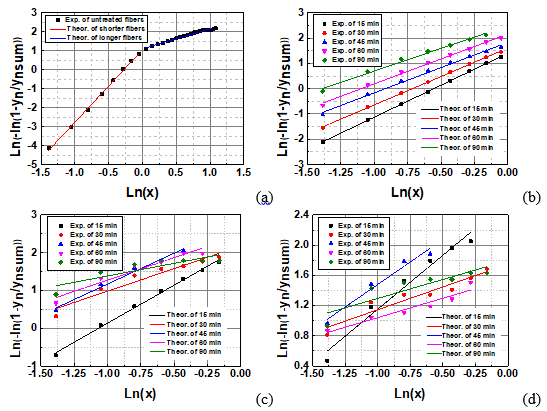Fig. 2. Exponential fitting to number-weighted length distribution of (a) untreated cellulose fibers and treated cellulose fibers with sulfuric acid hydrolysis at (b) 50 °C, (c) 60 °C, and (d) 70 °C

Similar conclusions can be obtained from Figs. 2(c) and (d), as well as Table S3, and S4. It can also be concluded from Fig. 2 that the number-weighted length distributions of treated cellulose with sulfuric acid hydrolysis at 50 °C, 60 °C, and 70 °C well fit the theoretical lines measured by exponential law; however, the degree of suitability between them was reduced as the reaction temperature increased.

Based on the discussion above, was concluded that the exponential law was more suitable for describing cellulose fiber length distribution in a large-scaled size range (e.g., 0.2 μm to 1 μm). Golombek and Rapp (1997) studied the rock particle size distribution on Mars using an exponential law, and found that the exponential law fit the particle size distribution well, which was smaller than 0.2 m. The degree of suitability, however, was reduced when the rock particles were in the 0.2 m to 0.4 m range.

Rosin-Rammler Fitting to Cumulative Mass-weighted Length Distribution of Cellulose Fibers

Figure 3 shows the R-R fitting to the mass-weighted length distribution of cellulose fibers, according to Eqs. 4 and 5. The fitting results shown in Fig. 3 were also derived from Tables S1, S2, S3, and S4 in the Supporting Information. Figure 3(a) shows the R-R fitting curve to mass-weighted length distribution of untreated cellulose fibers. It shows the cellulose length of 1 μm (ln(x) = 0) as the break point dividing the two straight lines derived from the R-R law. It was indicated that both the longer part (≥ 1 mm) and the shorter part (≤ 1 mm) of the untreated cellulose fibers fit the R-R distribution well, which corresponded with the results of Fig. 1(a) and Table S1. It was concluded that the fiber length of untreated cellulose obeyed the R-R distribution and it was estimated via an R-R curve.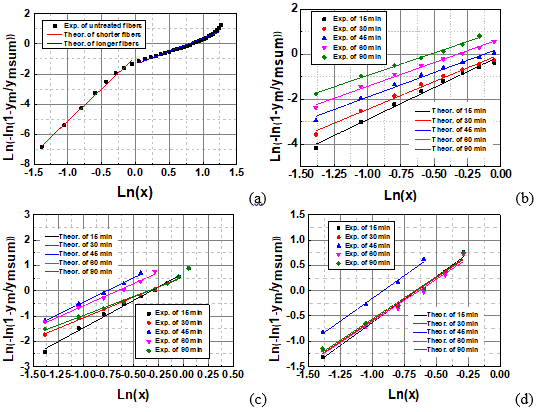Fig. 3. Rosin-Rammler fitting to mass-weighted length distribution of (a) untreated cellulose fibers and treated cellulose fibers with sulfuric acid hydrolysis at (b) 50 °C, (c) 60 °C, and (d) 70 °C

Figure 3(b) shows the R-R fitting to mass-weighted length distribution of treated cellulose fibers with sulfuric acid hydrolysis at 50 °C. Most of the lengths of treated cellulose fibers were less than 1 μm, as shown in Fig. 1(b). It was noted in Fig. 3(b) that the mass-weighted length distribution (experimental data) fit the theoretical lines prescribed by the R-R law well, which indicated that it was reasonable to estimate the cellulose fiber length distribution using the R-R law. As shown in Table S2 and Fig. 3(b), the values of R2 decreased from 0.9917 to 0.9859 as the acid hydrolysis duration increased from 15 min to 45 min, and then increased to 0.9941 as the duration increased to 90 min, which indicated that as the acid hydrolysis reaction progressed, the degree of suitability between R-R law and the fiber length distribution was reduced first and then increased, which was different from those of exponential law.

Similar conclusions were obtained from Figs. 3(c) and (d), as well as Table S3 and S4. It was also concluded from Fig. 3 that the mass-weighted length distributions of treated cellulose with sulfuric acid hydrolysis at 50 °C, 60 °C, and 70 °C fit the theoretical lines measured by the R-R law well; however, the degree of suitability between them decreased as the reaction temperature increased. However, the deviation degree of the R-R law was smaller than that of the exponential law.

Compared with exponential law (i.e., the cumulative number-weighted distribution), the R-R law (i.e., the cumulative mass-weighted distribution) showed a higher degree of suitability to the cellulose fiber length distribution after the acid treatment. Besides, this suitability provided some consideration for exploring the fracture mechanism of the cellulose fiber in future studies. When the flaws in the fragmentation process are activated continuously and independently, a random Poisson process occurs. Interestingly, the Poisson point process yields the fragments distribution following the R-R law (Gillespie 1992; Sousa, Jr. et al. 2011; Asztalos et al. 2012). The relationship between exponential and R-R laws as well as the application of the two fitting functions to estimate the cellulose fiber distribution with different treatment conditions will be discussed in the future studies.

CONCLUSIONS

1. Two functions (exponential and Rosin-Rammler (R-R) laws) were introduced to estimate the cellulose fiber length distribution. The results showed that the two above laws were effective in estimating the cellulose fiber length distribution in cellulose hydrolysis processes. Both the exponential and R-R laws fit the cellulose fiber length distribution well after sulfuric acid hydrolysis.
2. The cumulative number distribution from Fiber Tester fit the exponential distribution well, while the cumulative mass distribution obtained from the acid hydrolysis was well-fitted to the R-R law via an approximation process. The reduction of cellulose fiber length during the sulfuric acid hydrolysis weakened the suitability of exponential and R-R laws. The R-R law was more suitable than exponential laws, especially for the cellulose fiber length distribution in a small-scaled size range.
3. The results of this study suggested that the R-R law is a feasible way to estimate the cellulose fiber size distribution; this method has the potential to be applied to biorefining, cellulose nanocrystals preparation, among other things.

ACKNOWLEDGMENTS

Financial support for this project was provided by the National Key Research and Development Plan (Grant No.2017YFB0307901) and the National Nature Science Foundation of China (NSFC, No.21576213, 31270631).

REFERENCES CITED

Asztalos, A., Daniels, M., Sethi, A., Shen, T., Langan, P., Redondo, A., and Gnanakaran, S. (2012). “A coarse-grained model for synergistic action of multiple enzymes on cellulose,” Biotechnol. Biofuels 5(1), 1-15. DOI: 10.1186/1754-6834-5-55

Azzouz, B., Hassen, M. B., and Sakli, F. (2008). “Adjustment of cotton fiber length by the statistical normal distribution: Application to binary blends,” J. Eng. Fiber. Fabr. 3(3), 35-46.

Barbash, V. A., Yaschenko, O. V., Alushkin, S. V., Kondratyuk, A. S., Posudievsky, O. Y., and Koshechko, V. G. (2016). “The effect of mechanochemical treatment of the cellulose on characteristics of nanocellulose films,” Nanoscale Res. Lett. 11, 410. DOI: 10.1186/s11671-016-1632-1

Chang, C. P., Wang, I. C., Hung, K. J., and Perng, Y. S. (2010) “Preparation and characterization of nanocrystalline cellulose by acid hydrolysis of cotton linter,” Taiwan Journal of Forest Science 25(3), 231-244.

Dussán, K. J., Silva, D. D. V., Moraes, E. J. C., Arruda, P. V., and Felipe, M. G. A. (2014). “Dilute-acid hydrolysis of cellulose to glucose from sugarcane bagasse,” Chem. Engineer. Trans.38, 433-438. DOI: 10.3303/CET1438073

Elek, P., and Jaramaz, S. (2005). “Modeling of fragmentation of rapidly expanding cylinders,” Theor. Appl. 32(2), 113-130. DOI: 10.2298/TAM0502113E

Farizhandi, A. A. K., Zhao, H., and Lau, R. (2016). “Modeling the change in particle size distribution in a gas-solid fluidized bed due to particle attrition using a hybrid artificial neural network-genetic algorithm approach,” Chem. Eng. Sci. 155, 210-220. DOI: 10.1016/j.ces.2016.08.015

Fortunati, E., Puglia, D., Monti, M., Peponi, L., Santulli, C., Kenny, J. M., and Torre, L. (2013). “Extraction of cellulose nanocrystals from Phormium tenax fibres,” J. Polym. Environ.21(2), 319-328. DOI: 10.1007/s10924-012-0543-1

Gårdebjer, S., Bergstrand, A., Idström, A., Börstell, C., Naana, S., Nordstierna, L., and Larsson, A. (2015). “Solid-state NMR to quantify surface coverage and chain length of lactic acid modified cellulose nanocrystals, used as fillers in biodegradable composites,” Compos. Sci. Technol. 107, 1-9. DOI: 10.1016/j.compscitech.2014.11.014

Gillespie, D. T. (1992). “A rigorous derivation of the chemical master equation,” Physica A 188(1), 404-425. DOI: 10.1016/0378-4371(92)90283-V

Golombek, M., and Rapp, D. (1997). “Size-frequency distributions of rocks on Mars and Earth analog sites: Implications for future landed missions,” J. Geophys. Res.-Planet 102(E2), 4117-4129. DOI: 10.1029/96JE03319

Hertel, K. L., and Zervigon, M. G. (1936). “An optical method for the length analysis of cotton fibers,” Text. Res. J. 6(7), 331-339. DOI: 10.1177/004051753600600707

Krifa, M. (2008). “Fiber length distribution in cotton processing: A finite mixture distribution model,” Text. Res. J. 78(8), 688-698. DOI: 10.1177/0040517508083729

Kropholler, H. W., and Sampson, W. W. (2001). “The effect of fibers length distribution on suspension crowding,” J. Pulp Pap. Sci. 27(9), 301-305.

Kuang, X., Hu, Y., and Yu, C. (2015). “The theoretical yarn unevenness of cotton considering the joint influence of fiber length distribution and fiber fineness,” Text. Res. J. 86(2), 138-144. DOI: 10.1177/0040517515586161

Kwan, T. H., Ong, K. L., Haque, M. A., Kwan, W. H., Kulkarni, S., and Lin, C. S. K. (2018). “Valorisation of food and beverage waste via saccharification for sugars recovery,” Bioresource Technol. 255, 67-75. DOI: 10.1016/j.biortech.2018.01.077

Morris, N. J., and Warburton, B. (1984). “Particle size analysis of microcapsules,” J. Pharm. Pharmacol. 36(2), 73-76. DOI: 10.1111/j.2042-7158.1984.tb02997.x

Ring, G. J. F., and Bacon, A. J. (1997). “Multiple component analysis of fiber length distributions,” TAPPI J. 78(7), 224-231.

Rosin, P., and Rammler, E. (1933). “The laws governing the fineness of powdered coal,” Journal of the Institute of Fuel 7, 29-36.

Sakdaronnarong, C., Pipathworapoom, W., Vichitsrikamol, T., Sema, T., Posoknistakul, P., Koo-amornpattana, W., and Laosiripojana, N. (2018). “Integrative process for a sugarcane bagasse biorefinery to produce glucose, bio-oil and carbon microspheres,” Process Saf. Environ. 116, 1-13. DOI: 10.1016/j.psep.2018.01.006

Smith, J. E., and Jordan, M. L. (1964). “Mathematical and graphical interpretation of the log-normal law for particle size distribution analysis,” Journal of Colloid Science 19(6), 549-559. DOI:10.1016/0095-8522(64)90069-8

Sousa, Jr., R., Carvalho, M. L., Giordano, R. L. C., and Giordano, R. C. (2011). “Recent trends in the modeling of cellulose hydrolysis,” Braz. J. Chem. Eng. 28(4), 545-564. DOI: 10.1590/S0104-66322011000400001

Tasman, J. E. (1972). “The fiber length of Bauer-McNett screen fractions,” TAPPI J. 55(1), 136-138.

Turcotte, D. L. (1986). “Fractals and fragmentation,” J. Geophys. Res.-Sol. Ea. 91(B2), 1921-1926. DOI: 10.1029/JB091iB02p01921

Wang, J., Niu, J., Sawada, T., Shao, Z., and Serizawa, T. (2017). “A bottom-up synthesis of vinyl-cellulose nanosheets and their nanocomposite hydrogels with enhanced strength,” Biomacromolecules 18(12), 4196-4205. DOI: 10.1021/acs.biomac.7b01224

Weibull, W. (1951). “A statistical distribution function of wide applicability,” J. Appl. Mech. 18, 293-297.

Wu, H., Chen, F., Liu, M., and Wang, J. (2017). “Preparation of microcrystalline cellulose by liquefaction of eucalyptus sawdust in ethylene glycol catalyzed by acidic ionic liquid,” BioResources 12(2), 3766-3777. DOI: 10.15376/biores.12.2.3766-3777

Yan, J. F. (1975). “A method for the interpretation of fiber length classification data,” TAPPI J. 58(8), 191-192.

Yin, Y., Zhao, L., Jiang, X., Wang, H., and Gao, W. (2017). “Synthesis of triazine derivative and its application in the modification of cellulose nanocrystals,” BioResources 12(4), 7427-7438. DOI: 10.15376/biores.12.4.7427-7438

Zeidman, M. I., Batra, S. K., and Sasser, P. E. (1991). “Determining short fiber content in cotton. Part I: Some theoretical fundamentals,” Text. Res. J. 61(1), 21-30. DOI: 10.1177/004051759106100103

Article submitted: March 8, 2018; Peer review completed: May 19, 2018; Revised version received: August 20, 2018; Accepted: August 21, 2018; Published: August 27, 2018.

DOI: 10.15376/biores.13.4.7792-7804

APPENDIX

SUPPLEMENTARY INFORMATION

Table S1. Fitting Parameters for Exponential and R-R Laws of the Control Cellulose Fibers Without Sulfuric Acid Hydrolysis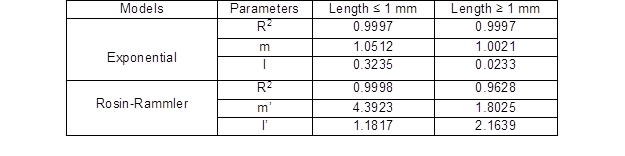Table S2. Fitting Parameters for Exponential and R-R Laws of Treated Cellulose Fibers with Sulfuric Acid Hydrolysis at 50 °C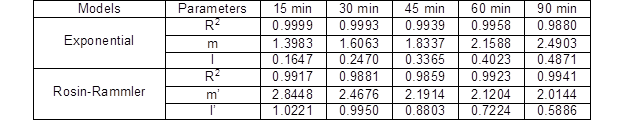Table S3. Fitting Parameters for Exponential and R-R Laws of Treated Cellulose Fibers with Sulfuric Acid Hydrolysis at 60 °C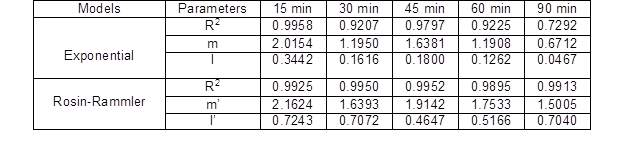Table S4. Fitting Parameters for Exponential and R-R Laws of Treated Cellulose Fibers with Sulfuric Acid Hydrolysis at 70 °C